Instant Maths Help:

# Edexcel A Level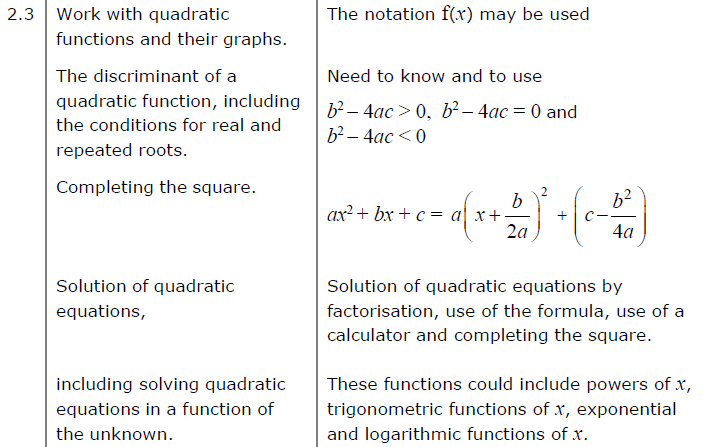##### Check solutions of quadratic equations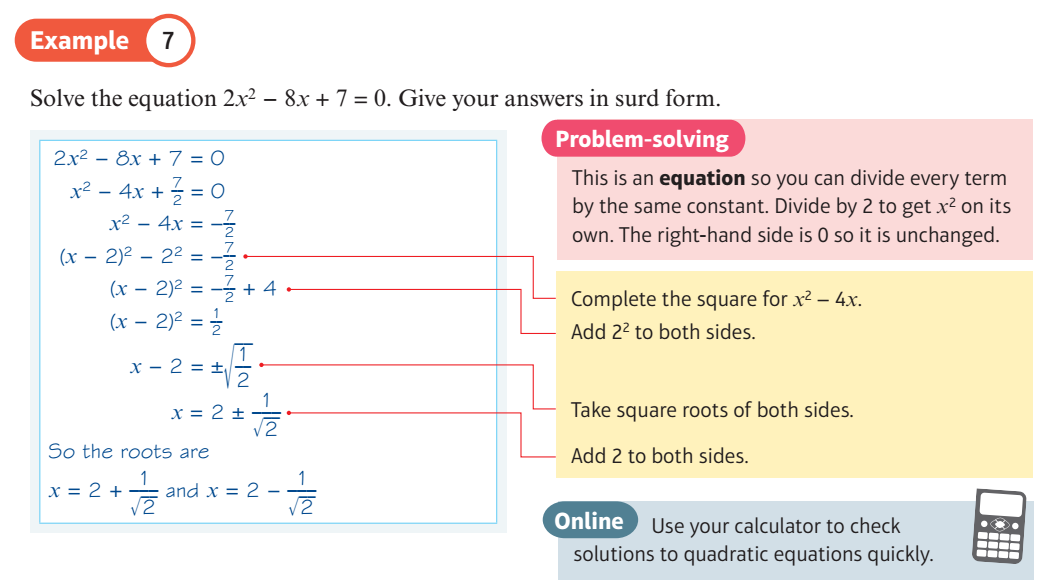##### Explore how the quadratic graph changes as the values of coefficients change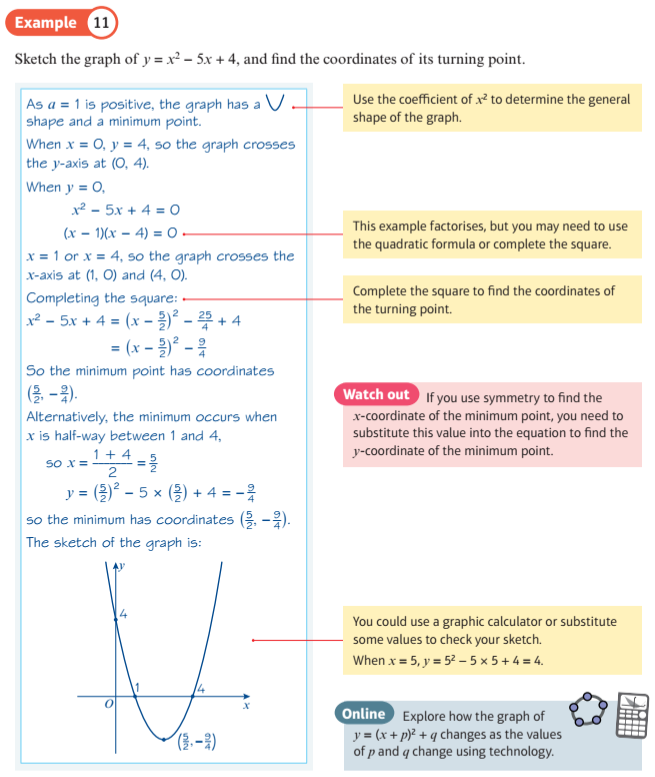##### Explore how the value of the discriminant changes with the value of the constant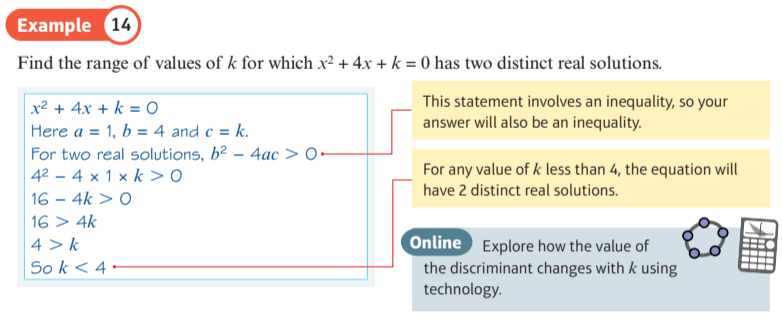##### Explore the trajectory of the spear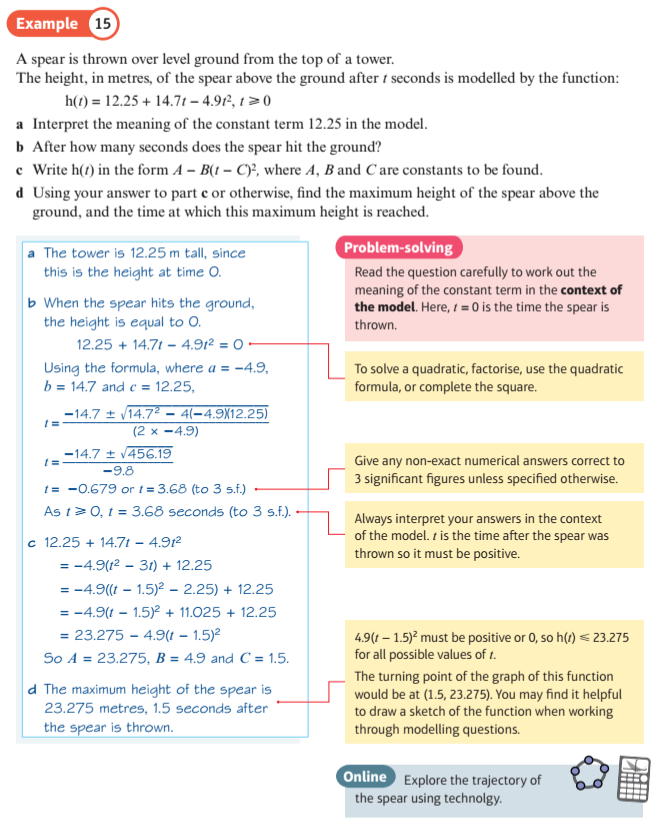Equations like ${{x^2} - 8x + 15 = 0}$ can be solved by plotting ${y = {x^2} - 8x + 15}$ and seeing where the curve crosses the x-axis.
Solve each of the equations in the display below. You can use the green point to help you by dragging it to the x-axis (where y = 0).
Using the sliders to match the blue dashed curve to the green curve will reveal the equation in each case, but can you say what the equation is before making the match?
Looking at where the green curve crosses the x-axis should help you.
Find the set of values of $k$ for which $x$ is real, given that $y = {x^2} + kx + (2k - 3) = 0$

(a) For what values of k does the equation have:
(i) equal roots
(ii) roots of the same sign
(iii) roots of opposite sign?

(b) What is the significance of the common point on all the graphs?

(c) What is the greatest minimum value that the expression y can have, and for what value of k does this occur?

(d) Using your answers to (a) (i), can you find a curve which passes through the minimum point on each curve? (Hint: think about transformations of functions.)